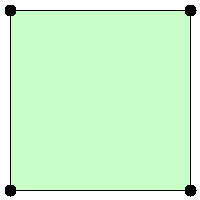##### Welcome to Subscribe On Youtube

Formatted question description: https://leetcode.ca/all/469.html

# 469. Convex Polygon

Medium

## Description

Given a list of points that form a polygon when joined sequentially, find if this polygon is convex (Convex polygon definition).

Note:

1. There are at least 3 and at most 10,000 points.
2. Coordinates are in the range -10,000 to 10,000.
3. You may assume the polygon formed by given points is always a simple polygon (Simple polygon definition). In other words, we ensure that exactly two edges intersect at each vertex, and that edges otherwise don’t intersect each other.

Example 1:

[[0,0],[0,1],[1,1],[1,0]]

Explanation:Example 2:

[[0,0],[0,10],[10,10],[10,0],[5,5]]

Explanation:## Solution

If a polygon is convex, then all interior angles are strictly less than 180 degrees. In other words, suppose there is an object traveling along the edges, starting from any point and finally going back to the starting point, then each time the object turns, it should turn in the same direction (always clockwise or always counterclockwise).

Suppose two edges have vectors (x1, y1) and (x2, y2) respectively, then the turning direction can be represented by x1 * y2 - x2 * y1. The positive result represents turning counterclockwise and the negative result represents turning clockwise.

If the turning direction is always the same, return true. Otherwise, return false.

• class Solution {
public boolean isConvex(List<List<Integer>> points) {
int edgesCount = points.size();
if (edgesCount < 3)
return false;
long cur = 0, pre = 0;
for (int i = 0; i < edgesCount; i++) {
int x1 = points.get((i + 1) % edgesCount).get(0) - points.get(i).get(0);
int x2 = points.get((i + 2) % edgesCount).get(0) - points.get(i).get(0);
int y1 = points.get((i + 1) % edgesCount).get(1) - points.get(i).get(1);
int y2 = points.get((i + 2) % edgesCount).get(1) - points.get(i).get(1);
cur = x1 * y2 - x2 * y1;
if (cur != 0 && cur * pre < 0)
return false;
if (cur != 0)
pre = cur;
}
return true;
}

}

• class Solution(object):
def isConvex(self, points):
"""
:type points: List[List[int]]
:rtype: bool
"""
calcDir = lambda x, y, z: (y - x) * (z - x) - (y - x) * (z - x)
pre = None
for i in range(0, len(points) - 2):
x = points[i]
y = points[i + 1]
z = points[i + 2]
c = calcDir(x, y, z)
if c == 0:
continue
if pre is None:
pre = c
if pre * c < 0:
return False
pre = c
if pre * calcDir(points[-1], points, points) < 0:
return False
if pre * calcDir(points[-2], points[-1], points) < 0:
return False
return True